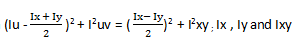Q.1
• a) ∑Fx=0
• b) ∑Fy=0
• c) ∑Fz=0
• d) ∑F≠0
Q.2
##### The assumptions for the calculations are done for the cable subjected to its own weight. In that one of the assumption is that the cable is ___________
• a) Extensible
• b) Non-flexible
• c) Flexible
• d) Static
Q.3
##### The magnitude of the resultant of the two vectors in calculations for cable subjected to its own weight is always:
• a) Greater than one of the vector’s magnitude
• b) Smaller than one of the vector’s magnitude
• c) Depends on the angle between them
• d) Axis we choose to calculate the magnitude
Q.4
• a) 900N
• b) 693N
• c) 646N
• d) 0N
Q.5
##### Cable subjected to its own weight takes a shape of a ____________ when is subjected to loadings.
• a) Helix
• b) Line
• c) Spring
• d) Complex figure
Q.6
• a) False
• b) True
Q.7
• a) 51.9cm
• b) 51.9mm
• c) 51.9m
• d) 5.19mm
Q.8
##### The assumptions for the calculations are done for the cable subjected to its own weight. In that one of the assumption is that the cable is flexible and the other is that the cable is ___________• a) Extensible
• b) Non-flexible
• c) Inextensible
• d) Static
Q.9
##### Due to which property the cable subjected to its own weight, it offers no resistance to bending?
• a) Extensible property
• b) Non-flexible property
• c) Flexibility property
• d) Static property
Q.10
##### The tensile force acting on the cable subjected to its own weight is in which direction w.r.t the cable?
• a) Perpendicular
• b) Parallel
• c) Tangential
• d) At an angle of 2 radians
Q.11
• a) False
• b) True
Q.12
##### The loading in the cable subjected to its own weight doesn’t change the ___________ of the cables.
• a) Geometry
• b) Colour
• c) Bending moment
• d) Point at which the shear stress is zero
Q.13
##### If the unknown variables in the calculations are more than the known quantities, then the number of equations required to solve all the unknown variables are for cable subjected to its own weight?
• a) Infinite
• b) Finite
• c) Not possible
• d) Question fault
Q.14
##### For calculations involved for cable subjected to its own weight all the vectors quantities obey :
• a) Parallelogram law of addition
• b) Parallelogram law of multiplication
• c) Parallelogram law of addition of square root of their magnitudes
• d) Parallelogram law of addition of square of their magnitudes
Q.15
##### If two equal vector forces are mutually perpendicular in the cable subjected to its own weight then the resultant force is acting at which angle as compared to one of the vector?
• a) 45 degree
• b) 90 degree
• c) 180 degree
• d) 0 degree